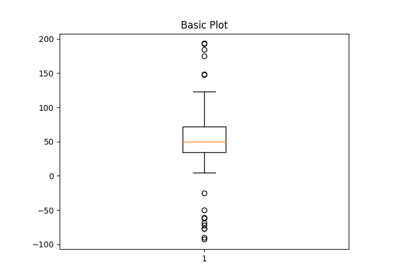# matplotlib.pyplot.boxplot¶

matplotlib.pyplot.boxplot(x, notch=None, sym=None, vert=None, whis=None, positions=None, widths=None, patch_artist=None, bootstrap=None, usermedians=None, conf_intervals=None, meanline=None, showmeans=None, showcaps=None, showbox=None, showfliers=None, boxprops=None, labels=None, flierprops=None, medianprops=None, meanprops=None, capprops=None, whiskerprops=None, manage_ticks=True, autorange=False, zorder=None, \*, data=None)[source]

Make a box and whisker plot.

Make a box and whisker plot for each column of x or each vector in sequence x. The box extends from the lower to upper quartile values of the data, with a line at the median. The whiskers extend from the box to show the range of the data. Flier points are those past the end of the whiskers.

Parameters: xArray or a sequence of vectors.The input data. notchbool, optional (False)If True, will produce a notched box plot. Otherwise, a rectangular boxplot is produced. The notches represent the confidence interval (CI) around the median. See the entry for the bootstrap parameter for information regarding how the locations of the notches are computed. Note In cases where the values of the CI are less than the lower quartile or greater than the upper quartile, the notches will extend beyond the box, giving it a distinctive "flipped" appearance. This is expected behavior and consistent with other statistical visualization packages. symstr, optionalThe default symbol for flier points. Enter an empty string ('') if you don't want to show fliers. If None, then the fliers default to 'b+' If you want more control use the flierprops kwarg. vertbool, optional (True)If True (default), makes the boxes vertical. If False, everything is drawn horizontally. whisfloat or (float, float) (default = 1.5)The position of the whiskers. If a float, the lower whisker is at the lowest datum above Q1 - whis*(Q3-Q1), and the upper whisker at the highest datum below Q3 + whis*(Q3-Q1), where Q1 and Q3 are the first and third quartiles. The default value of whis = 1.5 corresponds to Tukey's original definition of boxplots. If a pair of floats, they indicate the percentiles at which to draw the whiskers (e.g., (5, 95)). In particular, setting this to (0, 100) results in whiskers covering the whole range of the data. "range" is a deprecated synonym for (0, 100). In the edge case where Q1 == Q3, whis is automatically set to (0, 100) (cover the whole range of the data) if autorange is True. Beyond the whiskers, data are considered outliers and are plotted as individual points. bootstrapint, optionalSpecifies whether to bootstrap the confidence intervals around the median for notched boxplots. If bootstrap is None, no bootstrapping is performed, and notches are calculated using a Gaussian-based asymptotic approximation (see McGill, R., Tukey, J.W., and Larsen, W.A., 1978, and Kendall and Stuart, 1967). Otherwise, bootstrap specifies the number of times to bootstrap the median to determine its 95% confidence intervals. Values between 1000 and 10000 are recommended. usermediansarray-like, optionalAn array or sequence whose first dimension (or length) is compatible with x. This overrides the medians computed by matplotlib for each element of usermedians that is not None. When an element of usermedians is None, the median will be computed by matplotlib as normal. conf_intervalsarray-like, optionalArray or sequence whose first dimension (or length) is compatible with x and whose second dimension is 2. When the an element of conf_intervals is not None, the notch locations computed by matplotlib are overridden (provided notch is True). When an element of conf_intervals is None, the notches are computed by the method specified by the other kwargs (e.g., bootstrap). positionsarray-like, optionalSets the positions of the boxes. The ticks and limits are automatically set to match the positions. Defaults to range(1, N+1) where N is the number of boxes to be drawn. widthsscalar or array-likeSets the width of each box either with a scalar or a sequence. The default is 0.5, or 0.15*(distance between extreme positions), if that is smaller. patch_artistbool, optional (False)If False produces boxes with the Line2D artist. Otherwise, boxes and drawn with Patch artists. labelssequence, optionalLabels for each dataset. Length must be compatible with dimensions of x. manage_ticksbool, optional (True)If True, the tick locations and labels will be adjusted to match the boxplot positions. autorangebool, optional (False)When True and the data are distributed such that the 25th and 75th percentiles are equal, whis is set to (0, 100) such that the whisker ends are at the minimum and maximum of the data. meanlinebool, optional (False)If True (and showmeans is True), will try to render the mean as a line spanning the full width of the box according to meanprops (see below). Not recommended if shownotches is also True. Otherwise, means will be shown as points. zorderscalar, optional (None)Sets the zorder of the boxplot. resultdictA dictionary mapping each component of the boxplot to a list of the Line2D instances created. That dictionary has the following keys (assuming vertical boxplots): boxes: the main body of the boxplot showing the quartiles and the median's confidence intervals if enabled. medians: horizontal lines at the median of each box. whiskers: the vertical lines extending to the most extreme, non-outlier data points. caps: the horizontal lines at the ends of the whiskers. fliers: points representing data that extend beyond the whiskers (fliers). means: points or lines representing the means. showcapsbool, optional (True)Show the caps on the ends of whiskers. showboxbool, optional (True)Show the central box. showfliersbool, optional (True)Show the outliers beyond the caps. showmeansbool, optional (False)Show the arithmetic means. cappropsdict, optional (None)Specifies the style of the caps. boxpropsdict, optional (None)Specifies the style of the box. whiskerpropsdict, optional (None)Specifies the style of the whiskers. flierpropsdict, optional (None)Specifies the style of the fliers. medianpropsdict, optional (None)Specifies the style of the median. meanpropsdict, optional (None)Specifies the style of the mean.

Notes

Note

In addition to the above described arguments, this function can take a data keyword argument. If such a data argument is given, the following arguments are replaced by data[<arg>]:

• All positional and all keyword arguments.

Objects passed as data must support item access (data[<arg>]) and membership test (<arg> in data).

## Examples using matplotlib.pyplot.boxplot¶